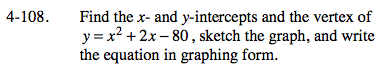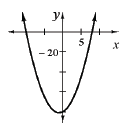### Home > A2C > Chapter Ch4 > Lesson 4.2.3 > Problem4-108

4-108.

Find the x- and y-intercepts and the vertex of y = x2 + 2x − 80, sketch the graph, and write the equation in graphing form. Homework Help ✎Find the x-intercepts and use them to locate the vertex.

y = (x + 1)2 − 81